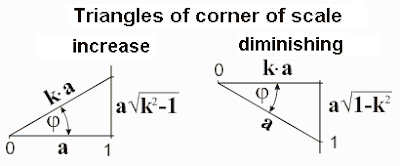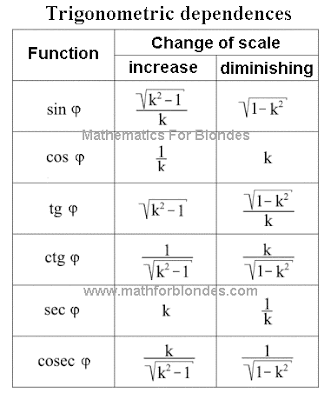## 10.26.2011

### Trigonometric dependences of corner of scale

We will consider trigonometric dependences of corner of scale in two rectangular triangles - at the increase of scale and at diminishing of scale.Trigonometric correlations of parties of the got rectangular triangles for diminishing and increase of scale we will take in a table.The got results can be compared to the relativistic radical from the theory of relativity of Einstein. Radicals in these equalizations very look like a sine and secant of diminishing of corner of scale. If to execute not difficult transformations, it is possible to get unit of measurement.The conducted transformations specify on that natural unit of measurement of speeds is speed of light. There are no grounds to suppose that there is a change of trigonometric dependences at passing through the point of symmetry of coefficient of scale. On it, it is possible to suppose that for faster-than-light speeds a relativistic radical takes on next values.On the basis of foregoing it is possible to suggest a next hypothesis about the structure of our Universe. Speed of set is a natural barrier separating slower-than-light part of Universe from faster-than-light part. Slower-than-light part of Universe we have possibility to look after. By virtue of specific properties of velocity of light, faster-than-light part of Universe can not be observed directly. It is possible to suppose that velocity of light is the axis of symmetry of distribution of substance in Universe. Dark matter and dark energy, that render affecting our part of Universe, can be in faster-than-light part of Universe.

If in Universe there are highly developed reasonable civilizations that captured faster-than-light technologies, then for an information transfer they will use not hertzian waves possessing velocity of light, and hard carriers of information on the basis of dark matter, transmissible with speeds, considerably excelling velocity of light.

If to equate trigonometric dependences of corner of scale with the values of trigonometric functions at 90° (it 1; 0 and 1/0), then for diminishing of scale they are taken to equality 0=1, for the increase of scale - to equality of k=0.

Our Universe has three limitations. In space by the border of universe явля-ется area, where velocity of light equals a zero. Outwardly our Universe is a point in space. Mathematical equalization of universe is equality 0=1 - any physical quantity with the unit of measurement in the scales of Universe equals a zero. This law of maintenance is confirmed by some researches of physicists, in particular, about it talked in the lecture of Andrei Linde.

Except spatial limitation, I exist limitations of speed. In slower-than-light part of Universe it is expressed in limitation long - distance between two positions of any point of space in time can not equal a zero. In physics this limitation it is accepted to name the absolute pitch of temperature. Faster-than-light part of Universe has limitation at times - time between two positions of any point in space can not equal a zero. The instantaneous moving in space without moving in time is impossible. Geometrically it can be expressed so: projection of speed on length and for a time can not equal a zero. Implementation of these terms is provided by the presence of rotation on the most different levels: atomic, planetary, galactic. It is possible to suppose that our Universe is similarly revolved in space.

More exact idea about principles of existence of Universe it is possible to get after the detailed study velocities of light as a physical process. For basis it is possible to accept position that velocity of light in our Universe is the result of co-operation of three dimension length with three dimension of time. Mathematically this co-operation is described by an multiplication. Physically our Universe has six dimension - three dimension of length and three dimension of time.

Expl for blondes: Now time to draw our Universe to look, what place she occupies in the space evolution.